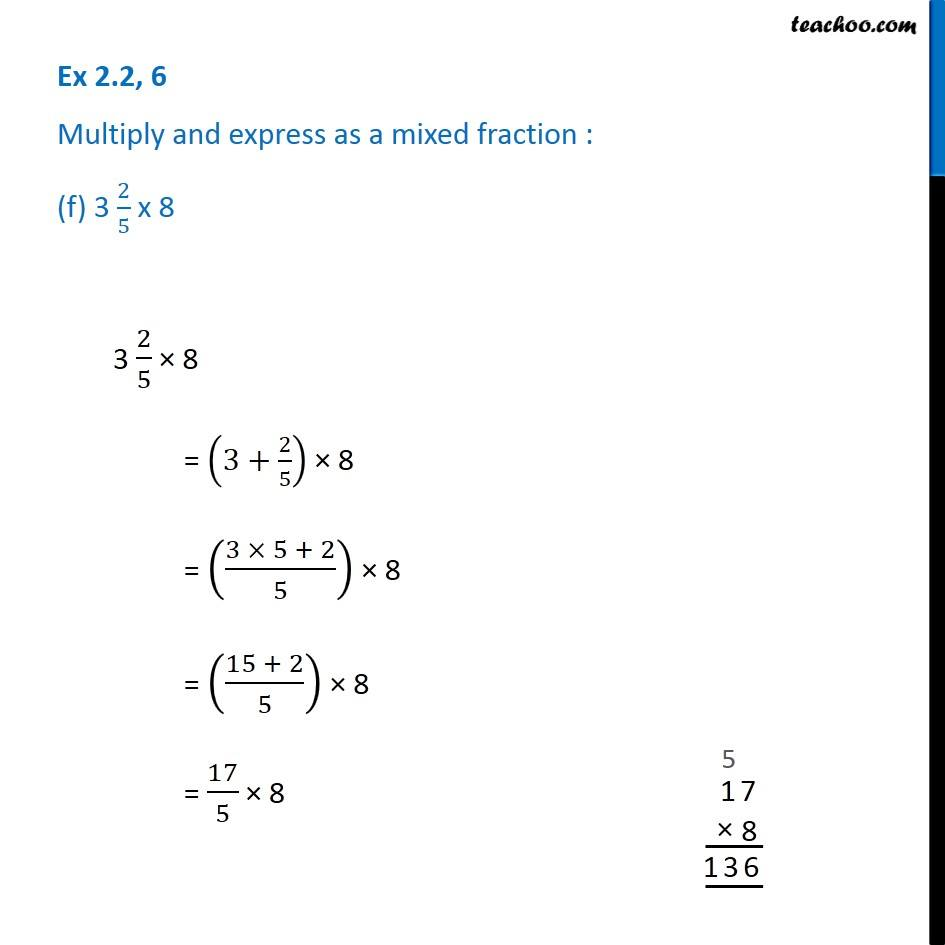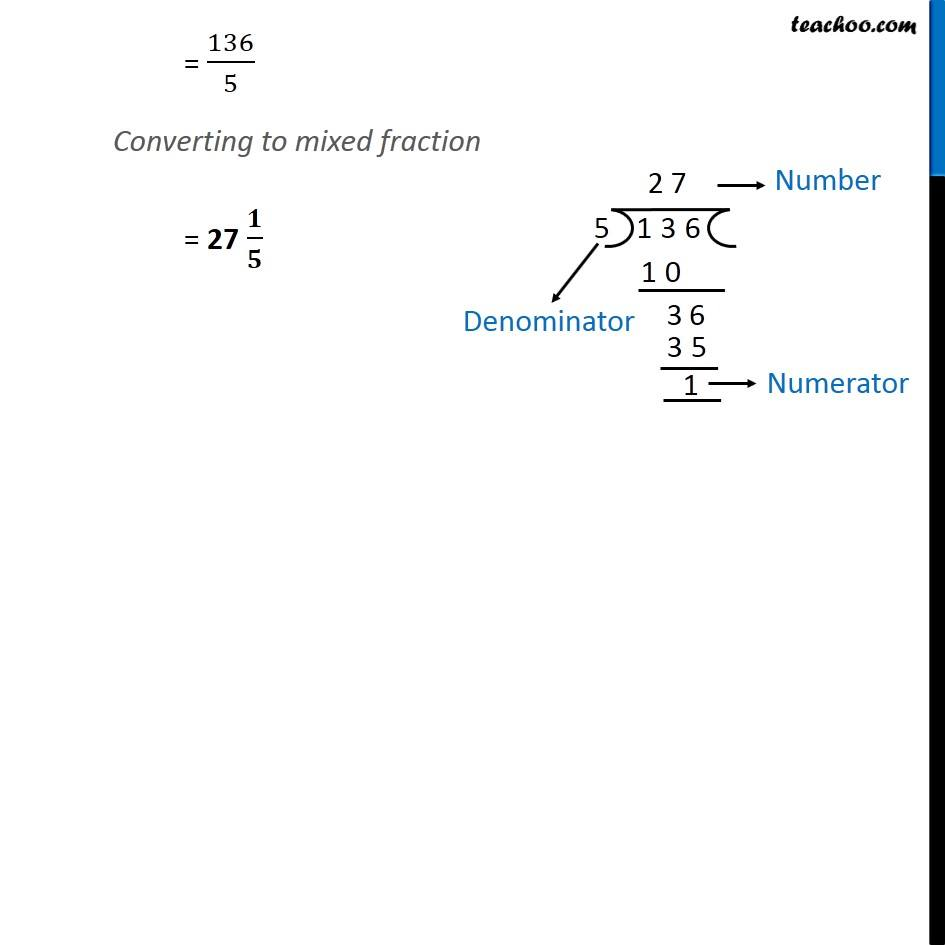1. Chapter 2 Class 7 Fractions and Decimals
2. Serial order wise
3. Ex 2.2

Transcript

Ex 2.2, 6 Multiply and express as a mixed fraction : (f) 3 2/5 x 83 2/5 × 8 = (3+2/5) × 8 = ((3 × 5 + 2)/5) × 8 = ((15 + 2)/5) × 8 = 17/5 × 8 = 136/5 Converting to mixed fraction = 27 𝟏/𝟓